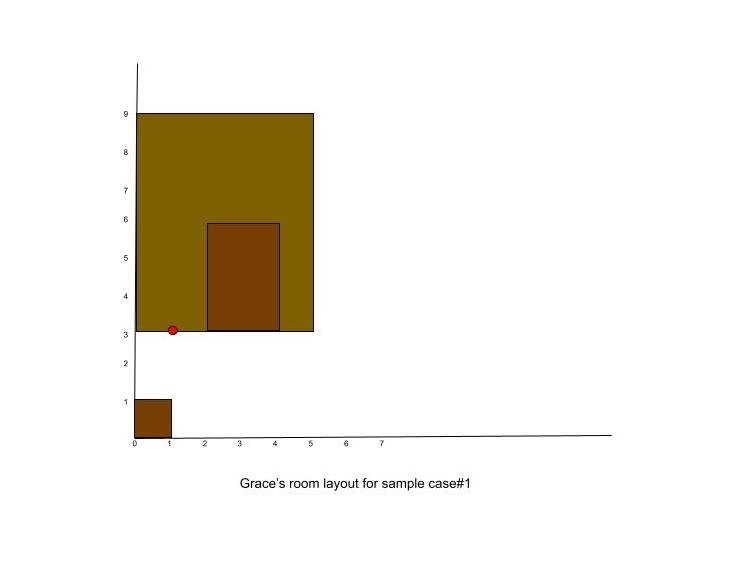시간 제한메모리 제한제출정답맞힌 사람정답 비율
서브태스크 참고 (추가 시간 없음) 1024 MB433100.000%

## 문제

With online classes in full swing, it is important for Grace to take breaks and keep herself hydrated at all times. She has decided to place a water bottle in her room in the most convenient place. This means that the position of this water bottle should be close to all the places in the room where she generally hangs out like the study desk, bed and coffee table among other places.

The room is represented in the form of a coordinate plane. The number of steps Grace needs to go from Point A to Point B is equal to the Manhattan distance between the 2 points. This means, Grace can only walk parallel to the axes of the coordinate plane and with each step, she can move one unit in either of the four directions.

Can you help her find a position in the room to keep the bottle, such that the sum of steps from the bottle to all her favourite furniture pieces will be minimum?

Notes:

• All the furniture (like study desk, bed, or coffee table) can be represented as rectangles of non-zero area in the plane with edges parallel to the axes.
• It is possible for furniture pieces to overlap, as she likes to work on her bed-table too.
• Assume that Grace can simply pass through the furniture while walking and does not need to go around them.

## 입력

The first line of the input gives the number of test cases, $T$. $T$ test cases follow.

The first line of each test case contains an integer $K$ which represents the number of objects in Grace's room.

$K$ lines follow, each of them describing one object. The $i$-th line contains four integers, $x_{i,1}$, $y_{i,1}$, $x_{i,2}$, $y_{i,2}$, where ($x_{i,1}$, $y_{i,1}$) represents coordinates of the bottom left corner and ($x_{i,2}$, $y_{i,2}$) represents coordinates of the top right corner of the $i$-th rectangular object.

## 출력

For each test case, output one line containing Case #i: x y, where $i$ is the test case number (starting from 1) and $x$ and $y$ are coordinates of the water bottle such that the sum of steps from these coordinates to all the furniture pieces will be minimum.

Note, the bottle can lie on the floor or on top of any furniture but should be placed on integer coordinates only.

If multiple solutions exist, output the one with minimum x coordinate, if multiple solutions have the same x coordinate output the one with minimum y coordinate.

## 제한

• $1 \le T \le 100$.
• $x_{i,1} < x_{i,2}$, for all $i$.
• $y_{i,1} < y_{i,2}$, for all $i$..

## Test Set 1 (7점)

시간 제한: 40 초

• $1 \le K \le 20$.
• $-100 ≤ x_{i,1}, y_{i,1}, x_{i,2}, y_{i,2} ≤ 100$, for all $i$.

## Test Set 2 (10점)

시간 제한: 90 초

• $1 \le K \le 10^5$.
• $-10^9 ≤ x_{i,1}, y_{i,1}, x_{i,2}, y_{i,2} ≤ 10^9$, for all $i$.

## 예제 입력 1

2
3
0 0 1 1
2 3 4 6
0 3 5 9
1
0 0 1 1


## 예제 출력 1

Case #1: 1 3
Case #2: 0 0In Sample Case #1, Grace can place the bottle at coordinates ($1$, $3$). It is at a distance of $2$ steps from first object, $1$ step from second and $0$ steps from the third one which gives us $3$ as the minimum possible sum of steps from a point.

In Sample Case #2, the water bottle can lie anywhere on the object itself but coordinates ($0$, $0$) correspond to the minimum x and y coordinates.

## 채점 및 기타 정보

• 예제는 채점하지 않는다.